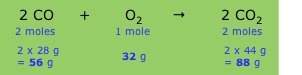# How can I know the relative number of grams of each substance used or produced with chemical equations?

Aug 26, 2014

You use the balanced chemical equation and the molar mass of each substance.

Consider the reaction

2C₂H₂ + 5O₂ → 4CO₂+ 2H₂O

Below each substance, write its coefficient and molar mass, like this:

2C₂H₂ + 5O₂ → 4CO₂+ 2H₂O
2×26.04 g + 5×32.00 g → 4×44.01 g + 2×18.02 g
52.08 g + 160.0 g → 176.0 g + 36.04 g

This tells you that 52.08 g of C₂H₂ react with 160.0 g of O₂ to form 176.0 g of CO₂ and 36.04 g of H₂O.

The relative numbers of grams are

C₂H₂ : O₂ : CO₂ : H₂O = 52.08 : 160.0 : 176.0 : 36.04

Here's another example: